Courses
Courses for Kids
Free study material
Offline Centres
More

# Polygons DiagonalsLast updated date: 08th Dec 2023
Total views: 302.4k
Views today: 3.02k## An Introduction

The diagonal of a polygon is a line segment that connects any two non-adjacent vertices. The number of diagonals and their attributes vary depending on the type of polygon and the number of sides. Let's review what a polygon is and what a diagonal is before learning the diagonal of a polygon formula.

A closed shape made up of three or more line segments is called a polygon. A line segment generated by joining any two non-adjacent vertices forms the diagonal of a polygon. Let's look at the formula for a polygon's diagonal, as well as some examples of solved problems. You can quickly count all of the possible diagonals of a basic polygon with a few sides. Counting polygons can be difficult when they become more intricate.

Fortunately, there is a straightforward formula for calculating the number of diagonals in a polygon. Because any vertex (corner) is connected to two other vertices by sides, those connections cannot be considered diagonals. That vertex, too, is unable to make a connection with itself.  As a result, ‘n’ we'll immediately lower the number of viable diagonals by three.

That vertex, too, is unable to make a connection with itself. For example, our door only has two diagonals if you don't include moving from the top hinge to the bottom opposite and back. Any solution will have to be divided by two.

### Diagonal- Polygons Diagonals

A diagonal is a segment of a polygon that connects two non-consecutive vertices. In a polygon, the number of diagonals that can be drawn from any vertex is three less than the number of sides. Multiply the number into totaling of diagonals per vertex (n - 3) by the number of vertices, n, then divide by 2 to get the total number of diagonals in a polygon (otherwise each diagonal is counted twice).

Number of Diagonals = $\frac{n(n-3)}{2}$

Simply subtract the total sides from the diagonals given by each vertex to another vertex to arrive at this formula. To put it another way, an n-sided polygon has n-vertices that can be connected in nC2 ways.

The formula obtained by subtracting n using nC2 methods is $\frac{n(n-3)}{2}$.

The total sides of a hexagon, for example, are six. As a result, the total diagonals are 6(6-3)/2 = 9

Let’s know what a diagonal is. A diagonal of a polygon can be defined as a line segment joining two vertices. From any given vertex, there are no diagonals to the vertex on either side of it, since that would lay on top of the side. Also,  remember that there is obviously no diagonal from a vertex back to itself. This means there are three less diagonals than the number of vertices. (We do not count diagonals to itself and one either side). This is a diagonal definition.

Here, we are going to discuss the number of diagonals in a polygon, diagonal definition.

### Formula for the Number of Diagonals

As described above, the number of diagonals from a single vertex is three less than the number of vertices or sides, or (n-3).

There are a total number of N vertices, which gives us n(n-3) diagonals.

But each diagonal of the polygon has two ends, so this would count each one twice. So as a final step we need to divide by 2, for the final formula:

Number of distinct diagonals = $\frac{n(n-3)}{2}$

where,

n  is the number of sides (or vertices).

### Diagonals of Polygon

The diagonals of a polygon is a segment line in which the ends are non-adjacent vertices of a polygon.

How many diagonals does n-polygon have? Let’s see the diagonals of a polygon and the no. of diagonals in a polygon.

For n = 3 we have a triangle. We can clearly see the triangle has no diagonals because each vertex has only adjacent vertices. Therefore, the number of diagonals in a polygon triangle is 0.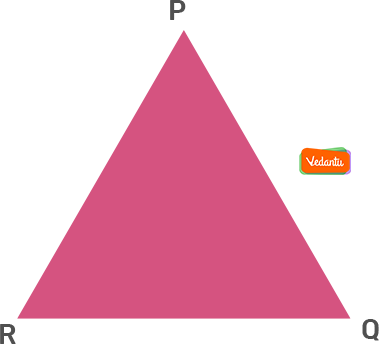For n = 4 we have quadrilateral.  It has 2 diagonals. Therefore, the number of diagonals in a polygon quadrilateral is 2.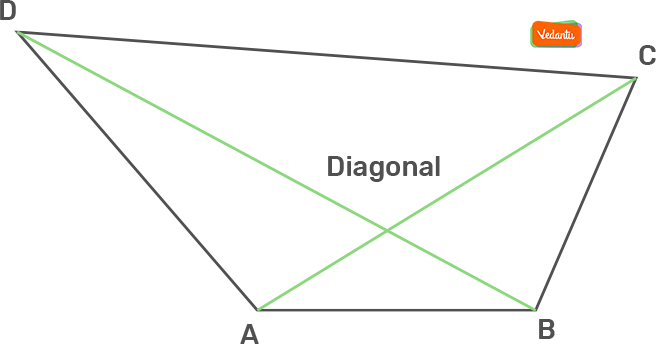For n = 5, we have a pentagon with 5 diagonals. Therefore, the number of diagonals in a polygon pentagon is 5.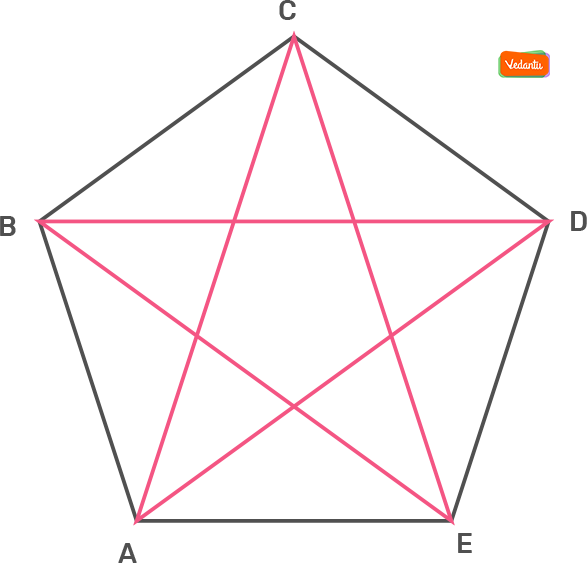For n = 6, n-polygon is called hexagon and it has 9 diagonals. Therefore, the number of diagonals in a polygon hexagon is 9.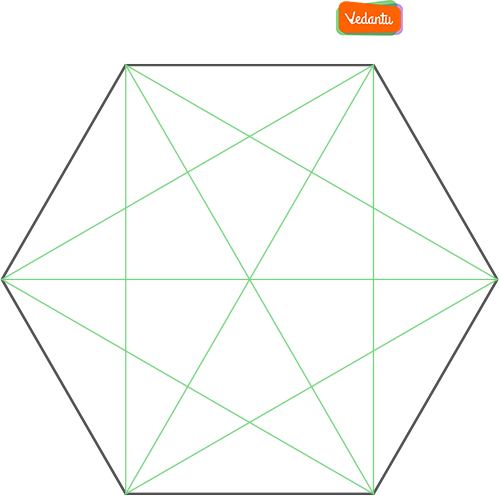Since n was a lower number we could easily draw the diagonals of n-polygons and then count the number of diagonals in a polygon.

### Diagonals in Real Life

Diagonals in rectangles, as well as diagonals in squares, add toughness to construction, whether for a house wall, bridge, or tall building. You may have seen diagonal wires used to keep the bridges steady. When houses are being built, look for the diagonal braces that tend to hold the walls straight and true.

Bookshelves and scaffolding are braced with diagonals. For a catcher in softball or for a catcher in baseball to throw out a runner at second base, the catcher throws along a diagonal from home plate to second.

The phone screen or computer screen you are viewing this lesson on is measured along its diagonal. A 21" screen never tells you the width and height of the screen; it is 21" from one corner to an opposite corner.

### Diagonal Formulas

1) Diagonal of a Rectangle Formula:

 Diagonal of a Rectangle = $\sqrt{l^{2} + b^{2}}$For rectangles, l is the length of the rectangle and b is the breadth of the rectangle.

2) Diagonal of a Square Formula:

Now let's look at a few different diagonal formulas to find the length of a diagonal of a square.

 Diagonal of a Square = $a\sqrt{2}$For squares, a is the side of a square

3) Diagonal of a Cube Formula:

For any given cube, we find the diagonal by using a three-dimensional version of the Pythagorean Theorem/distance formula:

 Diagonal of a cube = $\sqrt{s^{2} + s^{2} + s^{2}}$For cubes, s is the side of a cube

### Important Note

The above-given formula gives us the number of distinct diagonals - that is, the number of actual line segments. At times it is easy to miscount the diagonals of a polygon when doing it by eye.

If you glance quickly at the pentagon given below, you may be tempted to say that the number of diagonals is 10. After all, there are 2 at each vertex and 5 vertices. Few people watch them making 3 triangles, for 6 diagonals. But there are only 5 diagonals. You need to count them carefully.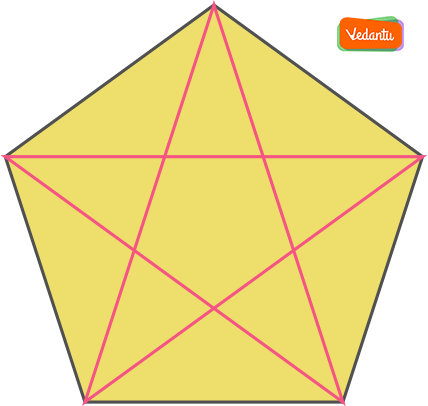### Solved Examples

Question 1) Find the total number of diagonals contained in an 11-sided regular polygon.

Solution) In an 11-sided polygon, total vertices are 11. Now, the 11 vertices can be joined with each other by C211 ways i.e. 55 ways.

Now, there are a total of 55 diagonals possible for an 11-sided polygon which includes its sides also. So, subtracting the sides will give the total number of diagonals contained by the polygon.

So, total diagonals contained within an 11-sided polygon = 55 -11 which is equal to 44.

Formula Method:

According to the formula, the number of diagonals equals $\frac{n(n-3)}{2}$.

So, 11-sided polygon will contain 11(11-3)/2 = 44 diagonals.

## FAQs on Polygons Diagonals

1. Which Polygon has Equal Diagonals?

A rhombus is a parallelogram with four equal sides. The diagonals of a rhombus are known to bisect each other and are perpendicular. A rectangle is a parallelogram with four 90° angles. The rectangle of any given rhombus bisect each other and have equal diagonals.

2. How Many Diagonals Does a Seven-Sided Polygon Have?

Classifying Polygons according to the number of diagonals.

 Polygon Name Number of Sides Number of Diagonals Quadrilateral 4 2 Pentagon 5 5 Hexagon 6 9 Heptagon 7 14

3. How do You Find the Number of Diagonals in a Polygon?

To find the total number of diagonals in a polygon, multiply the number of diagonals per vertex (n - 3) by the number of vertices, n, and divide by 2 (otherwise each diagonal is counted twice).

4. What is a 9 Sided Shape Called?

Enneagon: A nonagon is also known as an enneagon, which is derived from a Greek word called ‘enneagonon’, which means nine corners. The first use of the word nonagon dates back to the early seventeenth century, and has a Latin prefix that is (nona) and a Greek suffix (gon), making it a polygon with nine sides and nine angles.

5. What are polygons in Polygons Diagonals?

A simple figure bordered by straight lines is known as a polygon. In Greek, poly means numerous, and gon signifies angle. A triangle is the most basic polygon, with three sides and three angles totaling 180 degrees. This page contains the formula for a polygon's diagonal, as well as a discussion and examples.

Multiple-sided polygons can be regular (equal length and interior angles) or irregular (equal length and interior angles) (equal length and interior angles). A polygon can be classified as concave or convex based on its internal angles. If the inner angles are less than 180 degrees, the polygon is convex; otherwise, it is concave. It's worth mentioning that the sides of a polygon are always straight lines.

6. What are Polygon diagonal formulas?

A polygon is a closed shape made up of three or more line segments. The diagonal of a polygon is formed by a line segment formed by uniting any two non-adjacent vertices. There are no diagonals to the vertex on either side of it from any given vertex because it is on top of the side. Keep in mind that there is no diagonal between two vertex points. The number of diagonals is three less than the number of vertices, implying that there are three fewer diagonals than vertices.

7. What are diagonals in Polygons Diagonals?

A polygon is a closed-form that consists of three or more line segments. The diagonal of a polygon is formed by a line segment formed by connecting any two non-adjacent vertices. There are no diagonals to the vertex on either side of it from any vertex because that would be on top of the side. Keep in mind that from one vertex to the next, there is no diagonal. The number of diagonals is three fewer than the number of vertices, implying that there are three fewer diagonals than vertices.

8. Where can I get Polygons Diagonals - Formula, Diagonals, Solved Examples, and FAQs?

On Vedantu's website, you may find Polygons Diagonals - Formula, Diagonals, Solved Examples, and FAQs. There are full-fledged solved papers from previous years, significant problems, practice tests, and supplementary study material linked to Polygons Diagonals - Formula, Diagonals, Solved Examples. All of the information is available for free on Vedantu. You can use examples, solved papers, and other materials to help you understand your concepts better. Practice previous papers as well as key questions. If you have any doubts, you can consult our experts and participate in live sessions on Vedantu's learning platform.

9. What are the formulas in the chapter Polygons Diagonals?

Diagonals of polygons is an important chapter and it has formulas that are quite crucial. You now know how to get the diagonals of any polygon, what some real-world instances of diagonals are, and how to utilize the formula #of Diagonals =n(n3)2, where n is the polygon's number of sides (or vertices). We also went through how to find the length of a diagonal in cubes, squares, and rectangles using diagonal formulas.

Vedantu's website has a thorough explanation of each polygon's diagonal formula. It is available for free download and can be used to practice formulas